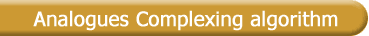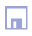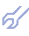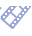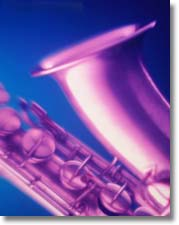When dispersion of noise is too big the application of non-parametric inductive selection algorithms is to be recommended. Analogues complexing algorithm should be used also in the case when number of variables exceed number of observations. The equal fuzziness of the model and object is reached automatically if the object itself is used for forecasting. This is done by searching analogues from the given data sample which are equivalent to the physical model. Forecasts are not calculated in the classical sense but selected from the table of observation data. If we succeed in finding for the last part of behavior trajectory (starting pattern), one or more analogous parts in the past (analogous pattern) the prediction can be achieved by applying the known continuation of these analogous patterns . The algorithm of selection of the analogous pattern has the following task: for the given output pattern PkA it is necessary to select the most similar patterns Pi,k+1 and to evaluate the forecast with the help of these patterns. The selection task is a four-dimensional problem with the following dimensions: set of variables used; number of analogues selected; width of the patterns (number of lines, used in each); values of weight coefficients with which patterns are complexed. As method of optimization the comparison of variants by accuracy criterion is used. To select similar patterns from all possible patterns in the time series, the following steps are developed: A. Reducing variable set size The choice of an optimal set of variables can be realised by preselection. It is necessary to identify a subset of effective variables, which were defined as the nucleus . B. Transformation of analogues As time-series may be non-stationary patterns with similar shapes may have different mean values, standard deviations and trends. In the literature, it is recommended to evaluate the difference between the process and its trend which is an unknown function of time. Another possibility gives the selection of differences where the criterion of stationarity is used as selection criterion. C. Selection of the most similar analogues The closest analogue is founded as the first analogue A1, the next one in distance A2 is called the second analogue and so on to the last analogue AF. Distances can be measured by means of the Euclidean distance of points of the output pattern and the analogue or by other measures of distance. D. Combining forecasts Every selected analogue has its continuation which gives a forecast. In such a way we obtain F forecasts, which are to combine. In the literature there are several principles for combination of forecasts, as weighted sum for example [8,17,20].